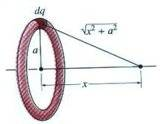# Calculate the electric potential and field

jlucas134
Here is the question. a hollow, thin walled insulating cylinder of radius b and height h has charge Q uniformly distributed over its surface. Calculate the electric potential and field at all points along the z axis of the tube.

Outside the tube
Inside the tube.

I know how to find the field, its just -"del" V, but my problem is finding V...

I know you have to take into account the area of the surface and the radius b...

here is what I have for the integral, which i don't know is right or not. Any help would be outstanding...If someone could help me set it up, I think i could get it from there.

(Q*k )/h * int (1/R), dz, limit from 0 to h, where R is equal to sqrt(b^2+(p-z)^2)

after integration

I get a

(Q*k )/h ln [(sqrt(b^2+(p-z)^2)+h-p)/(sqrt(b^2+p^2)-p)}

Reshma
I think you should divide your cylinder into elementary circular slabs. Find the expression for the potential at a point above the centre for one slab and integrate it for the entire length of the cylinder. Try and see if this works...

Last edited:
jlucas134
I tried it with no success..

I attempted to treat it like a ring of charge...finding E then integrating to find V.
Still no success.

I think I am getting lost in the "point any where on the axis inside or outside the tube".
any suggestions how to solve this?

Reshma
I tried it with no success..

I attempted to treat it like a ring of charge...finding E then integrating to find V.
Still no success.

I think I am getting lost in the "point any where on the axis inside or outside the tube".
any suggestions how to solve this?
The charge is distributed over the surface, so by Gauss's law the electric field inside the cylinder is zero. Find the value of the surface charge density $\sigma$ using Gauss's law for field outside the cylinder.The electric potential of a charged ring will be given by:
$$V = \frac{1}{4\pi \epsilon_0}\int_{ring} {dq \over r} = \frac{1}{4\pi \epsilon_0}\left(\frac{1}{\sqrt{a^2 + x^2}}\int dq\right)$$
a = h in your case.
Now write dq in terms of $\sigma$ and integrate along the z-axis.